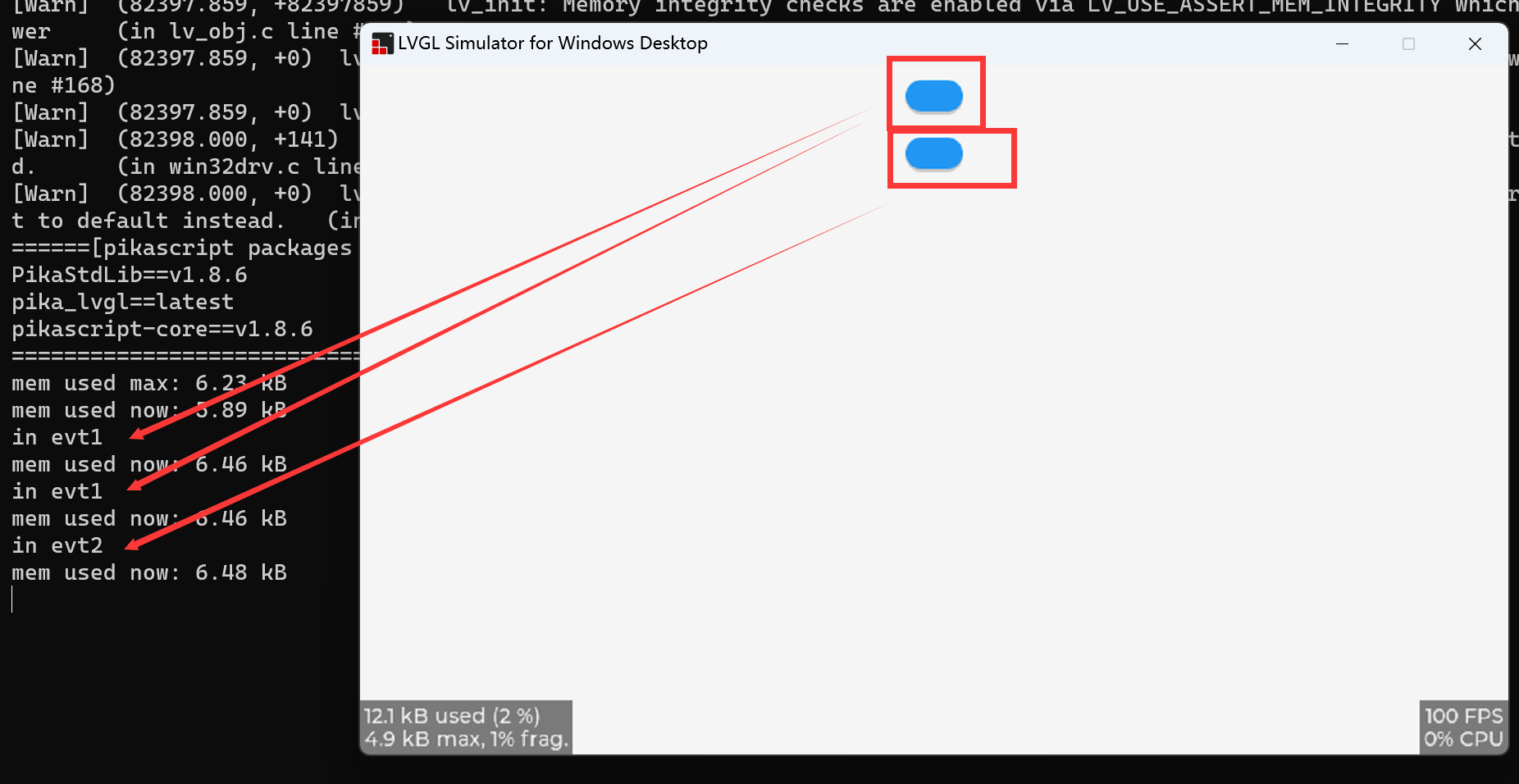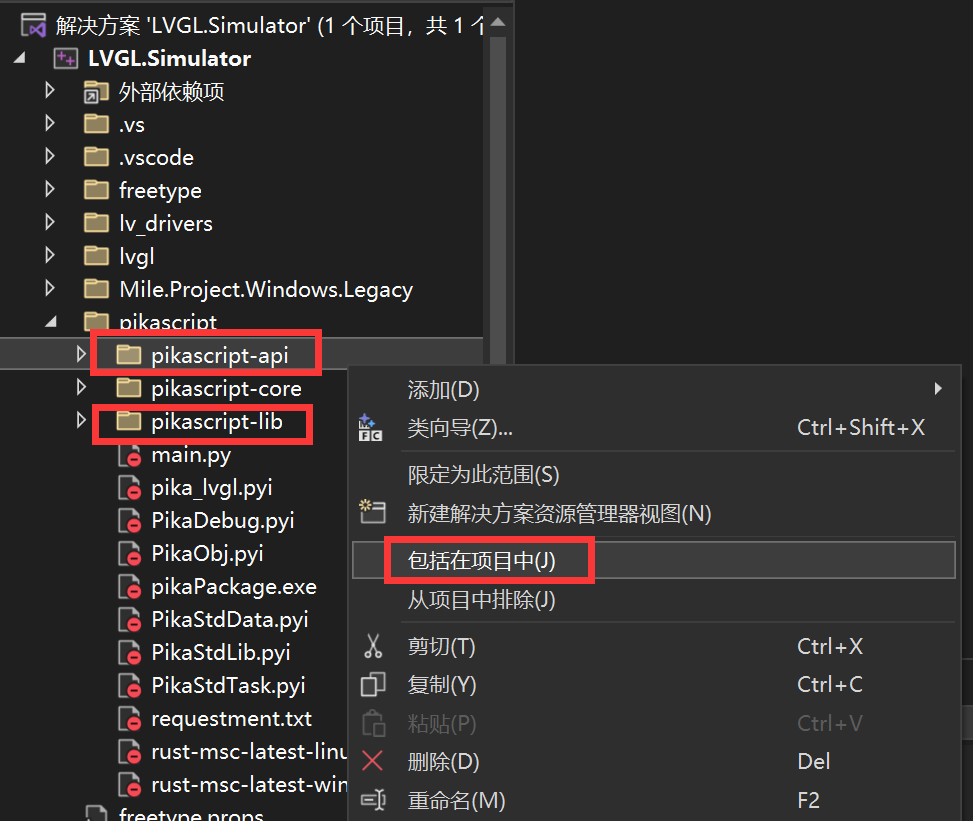The LVGL GUI Simulation Project provides an experimental environment for co-simulation of PikaPython and LVGL.

The GUI simulation can be performed on a PC using Visual Studio.

## 2.6.1. Get the project

http://pikascript.com/

Select lvgl-vs-simu, a Visual Studio simulation project, from the Project Builder on the official PikaPython website.

This project is branched from the official LVGL Visual Studio simulation project.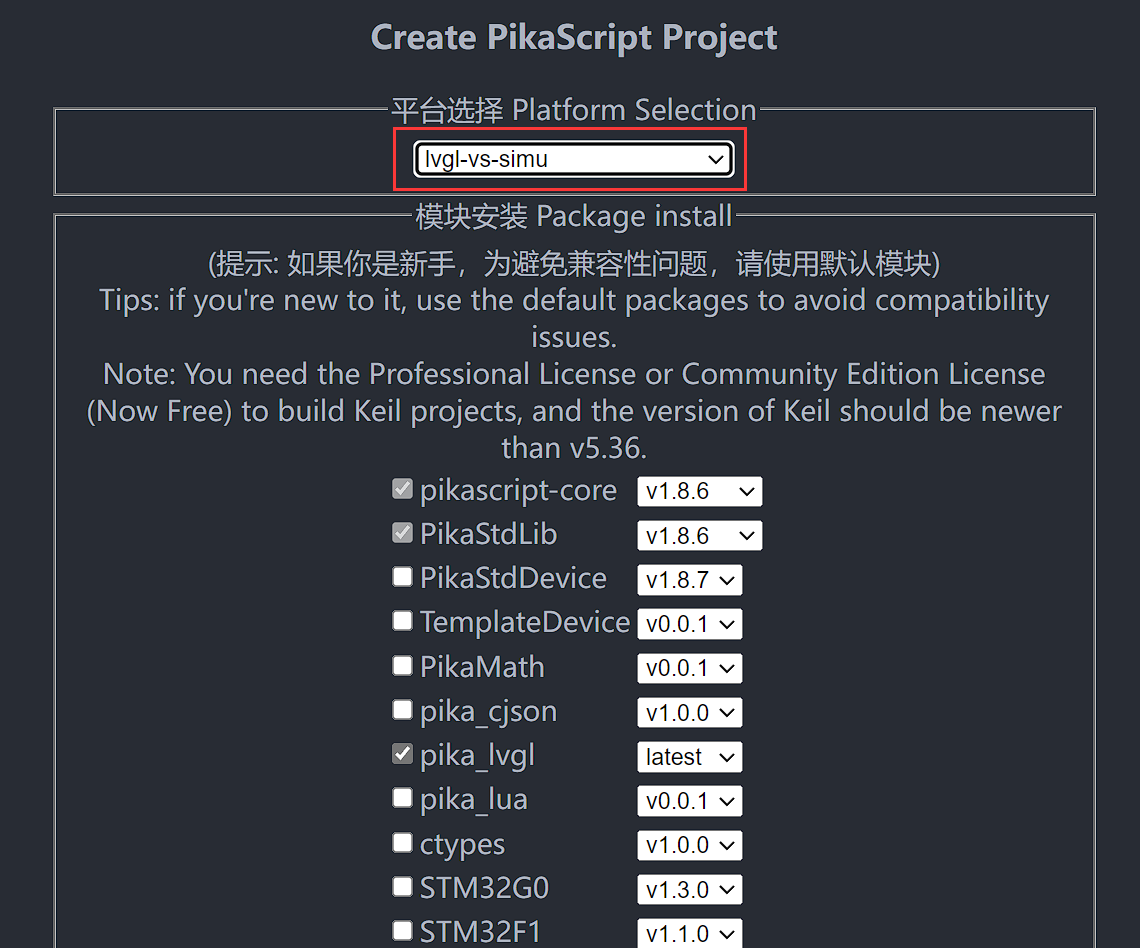Click Generate Project and wait about 1 minute.Unzip the project and open LVGL.Simulator.sln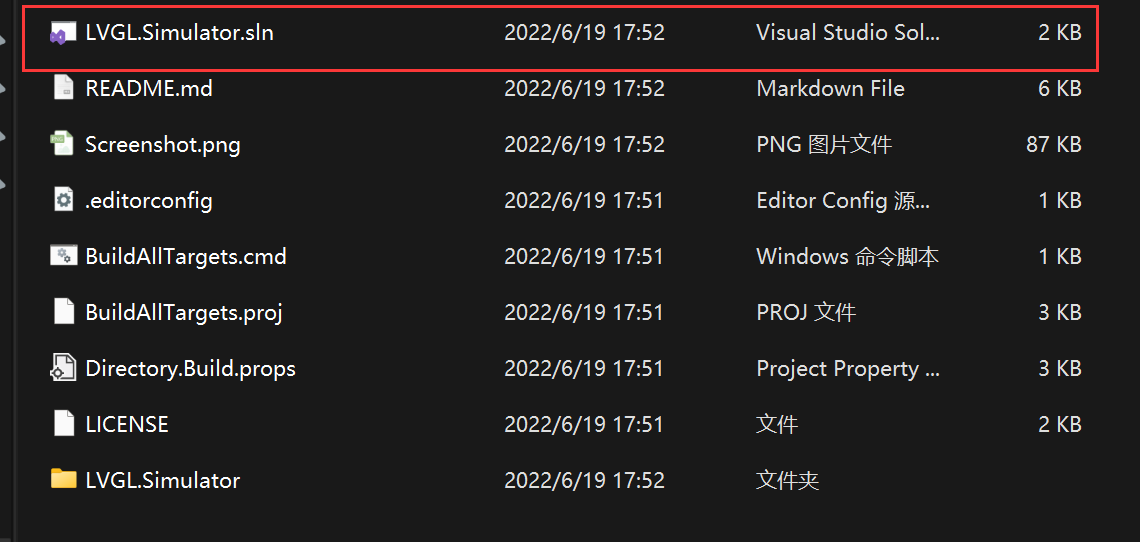Start compiling and running directly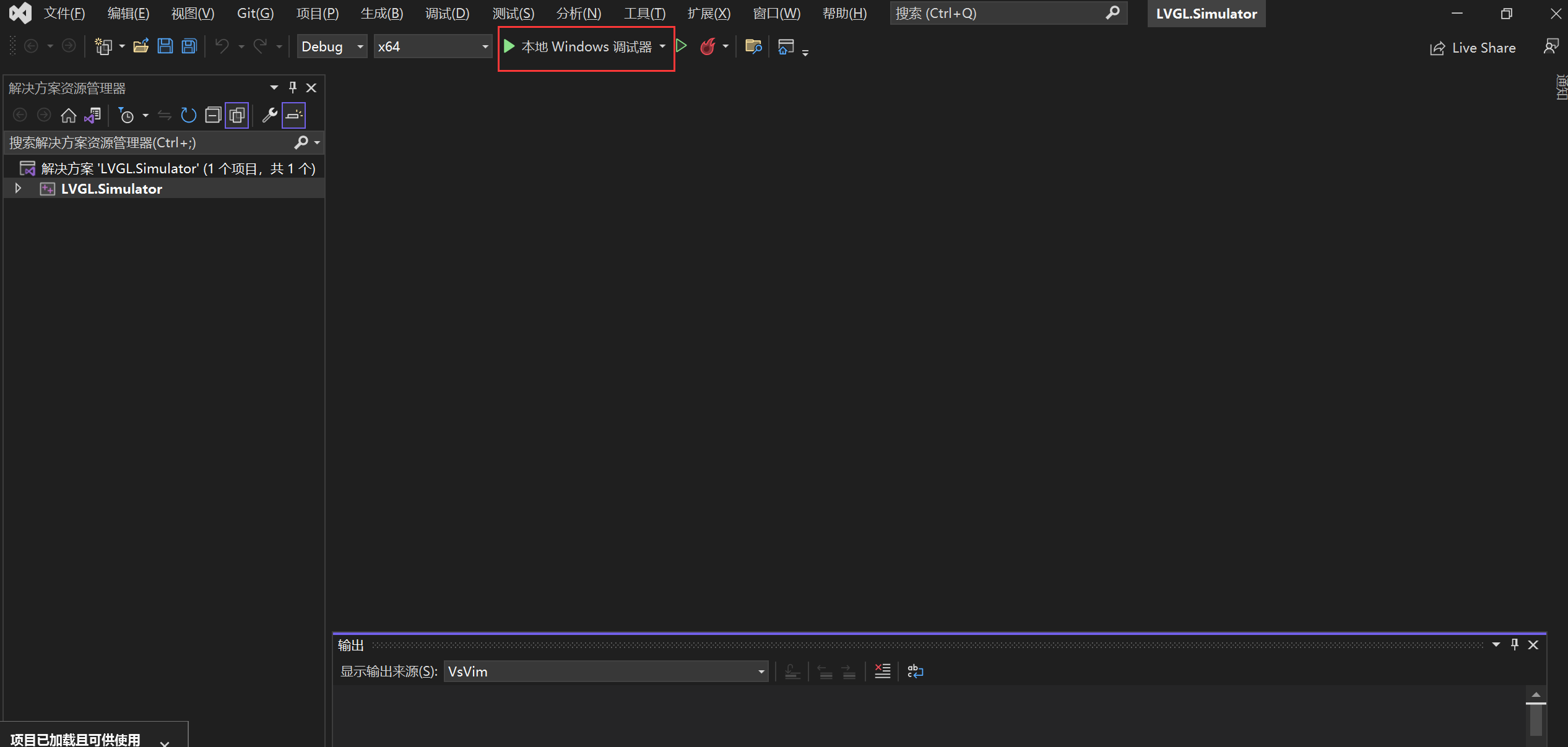You can see that the lvgl emulator has been successfully started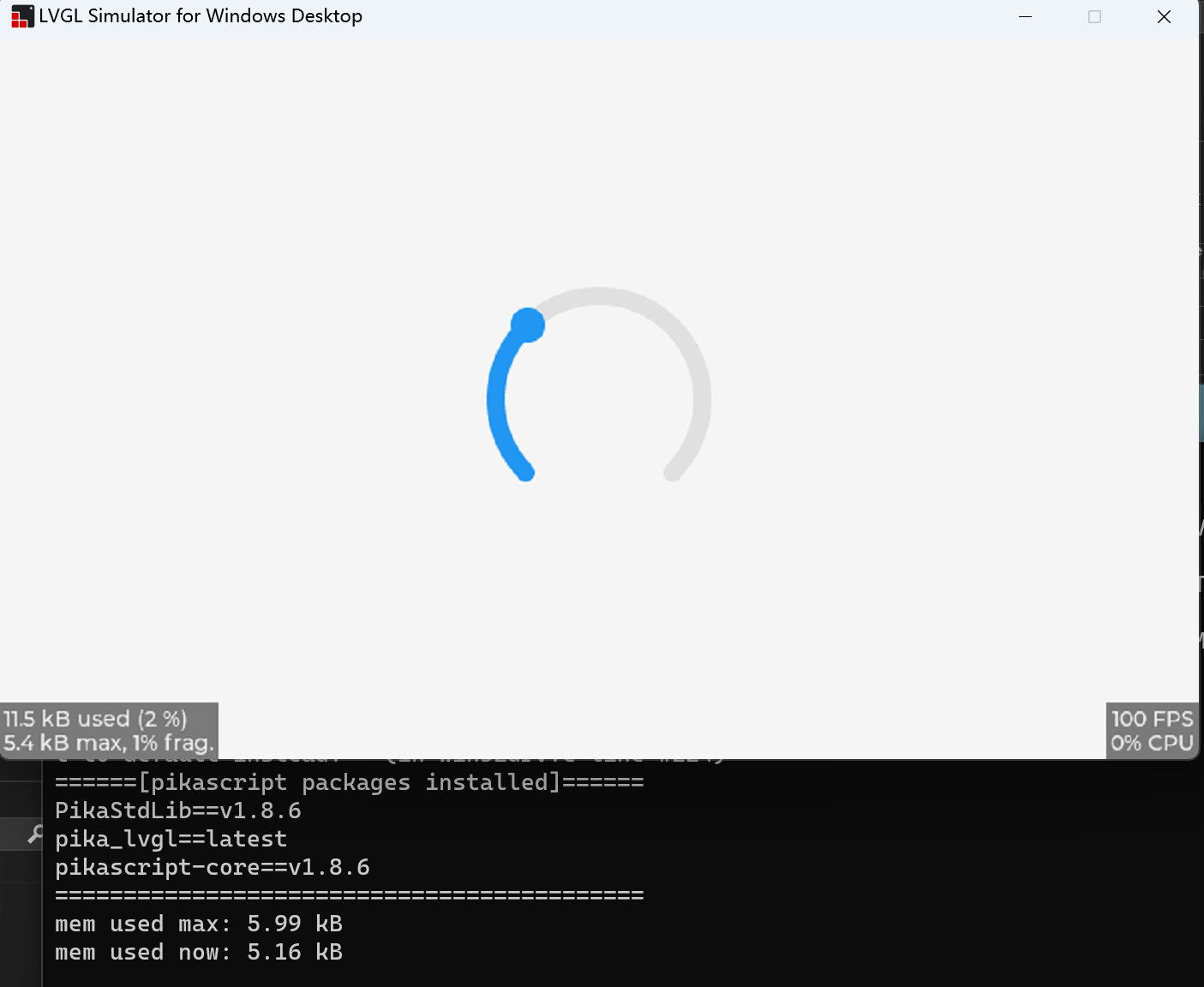## 2.6.2. Programming with Python

The Python file for running the project is in LVGL.Simulator/pikascript/main.py, and it is recommended to edit the Python file with VSCode.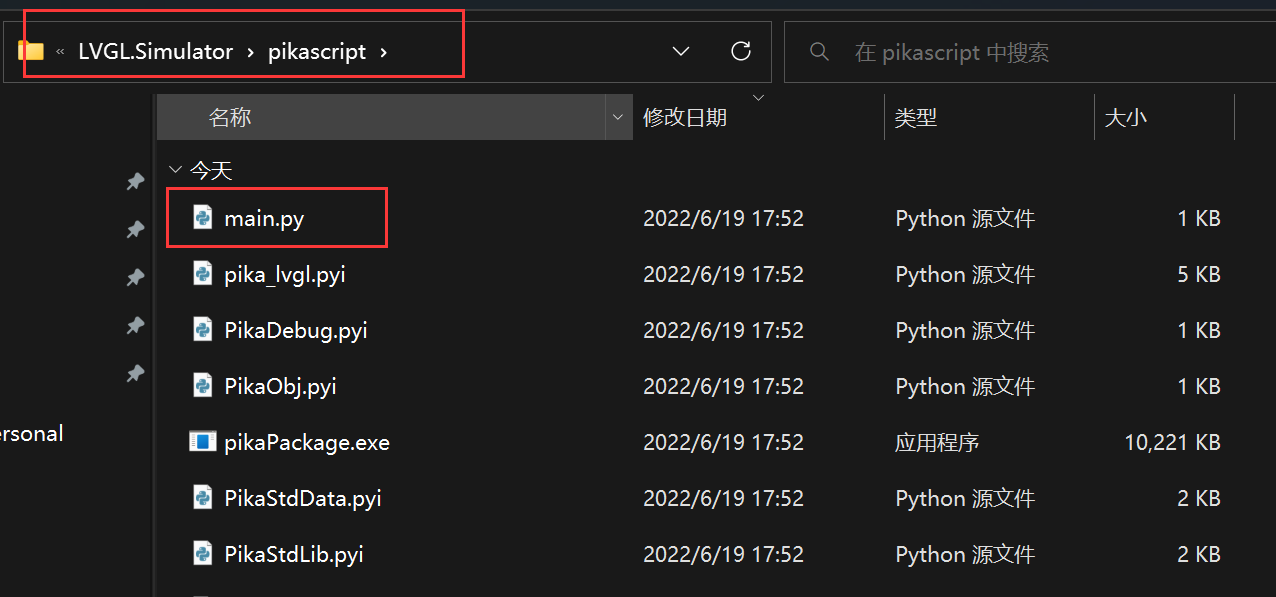The code in main.py is shown below, and the project will run this main.py when it starts

```# main.py
import pika_lvgl as lv
import PikaStdLib
mem = PikaStdLib.MemChecker()

# Create an Arc
arc = lv.arc(lv.scr_act())
arc.set_end_angle(200)
arc.set_size(150, 150)
arc.center()

print('mem used max: %0.2f kB' % (mem.getMax()))
print('mem used now: %0.2f kB' % (mem.getNow()))
```

More sample code

You can see more sample code in the /pikascript/examples/lvgl folder.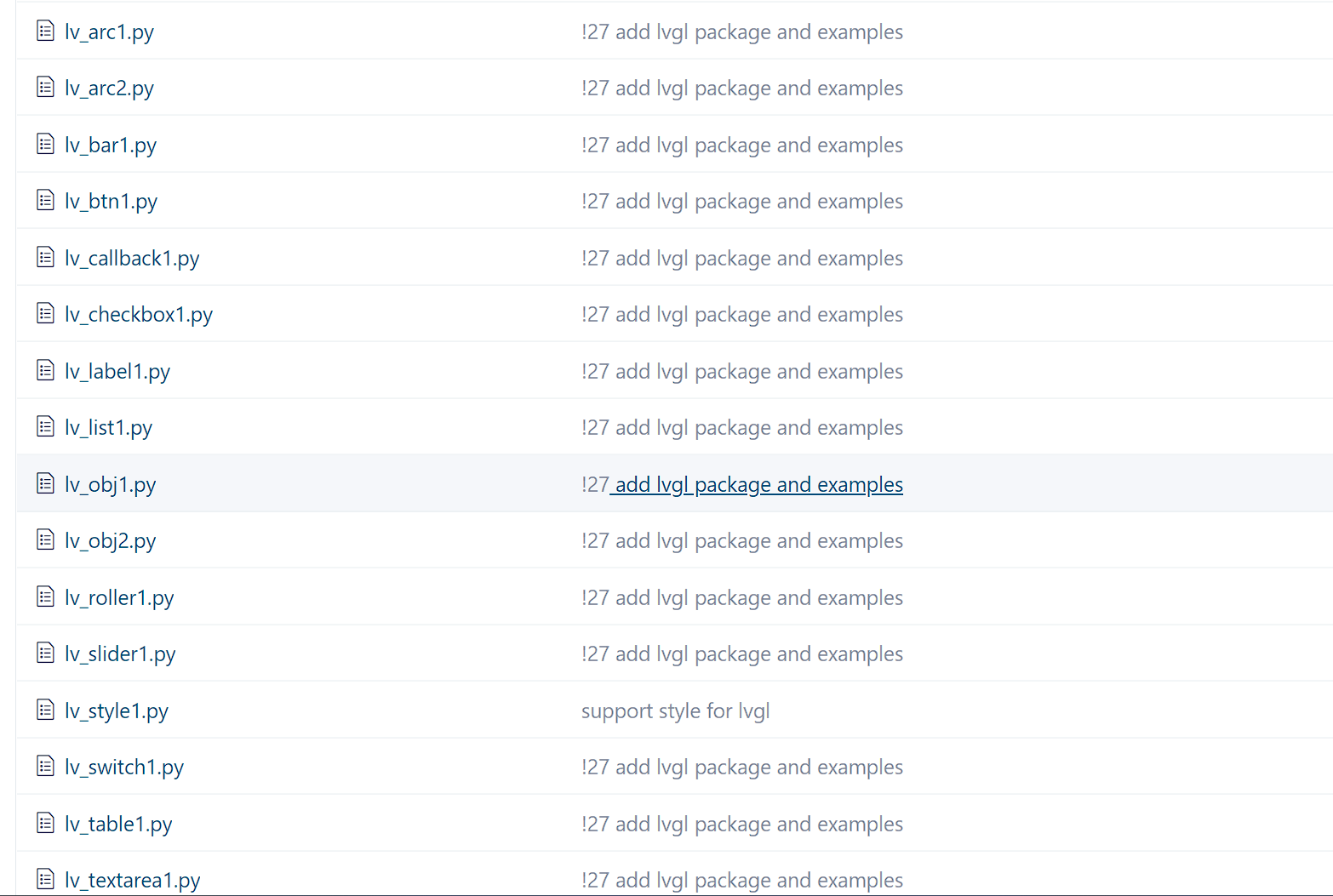For example, you can copy lv_callback1.py into main.py.

```# lv_callback1.py
import pika_lvgl as lv
import PikaStdLib
mem = PikaStdLib.MemChecker()

def event_cb_1(evt):
print('in evt1')
print('mem used now: %0.2f kB' % (mem.getNow()))

def event_cb_2(evt):
print('in evt2')
print('mem used now: %0.2f kB' % (mem.getNow()))

btn1 = lv.btn(lv.scr_act())
btn1.align(lv.ALIGN.TOP_MID, 0, 10)
btn2 = lv.btn(lv.scr_act())
btn2.align(lv.ALIGN.TOP_MID, 0, 50)

print('mem used max: %0.2f kB' % (mem.getMax()))
print('mem used now: %0.2f kB' % (mem.getNow()))
```

After replacing main.py, run PikaPython’s pre-compiler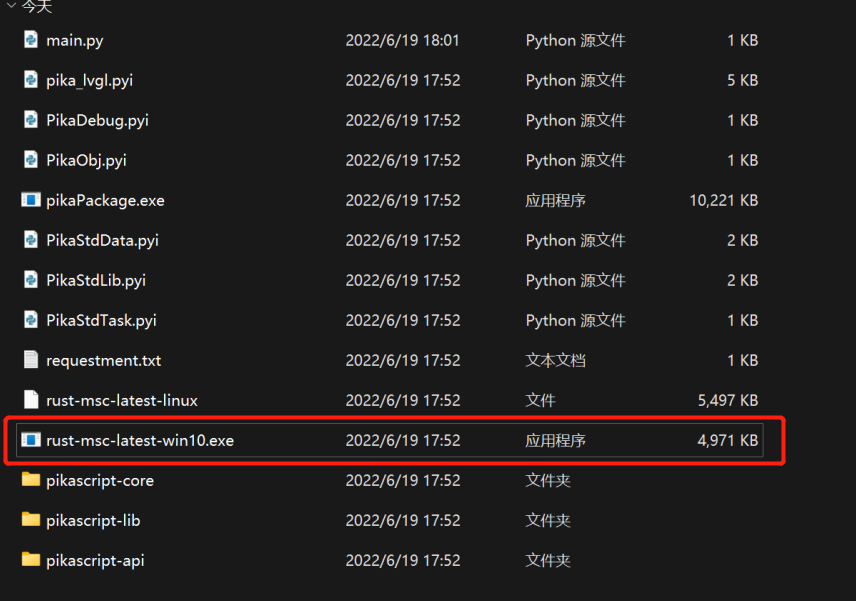and then start running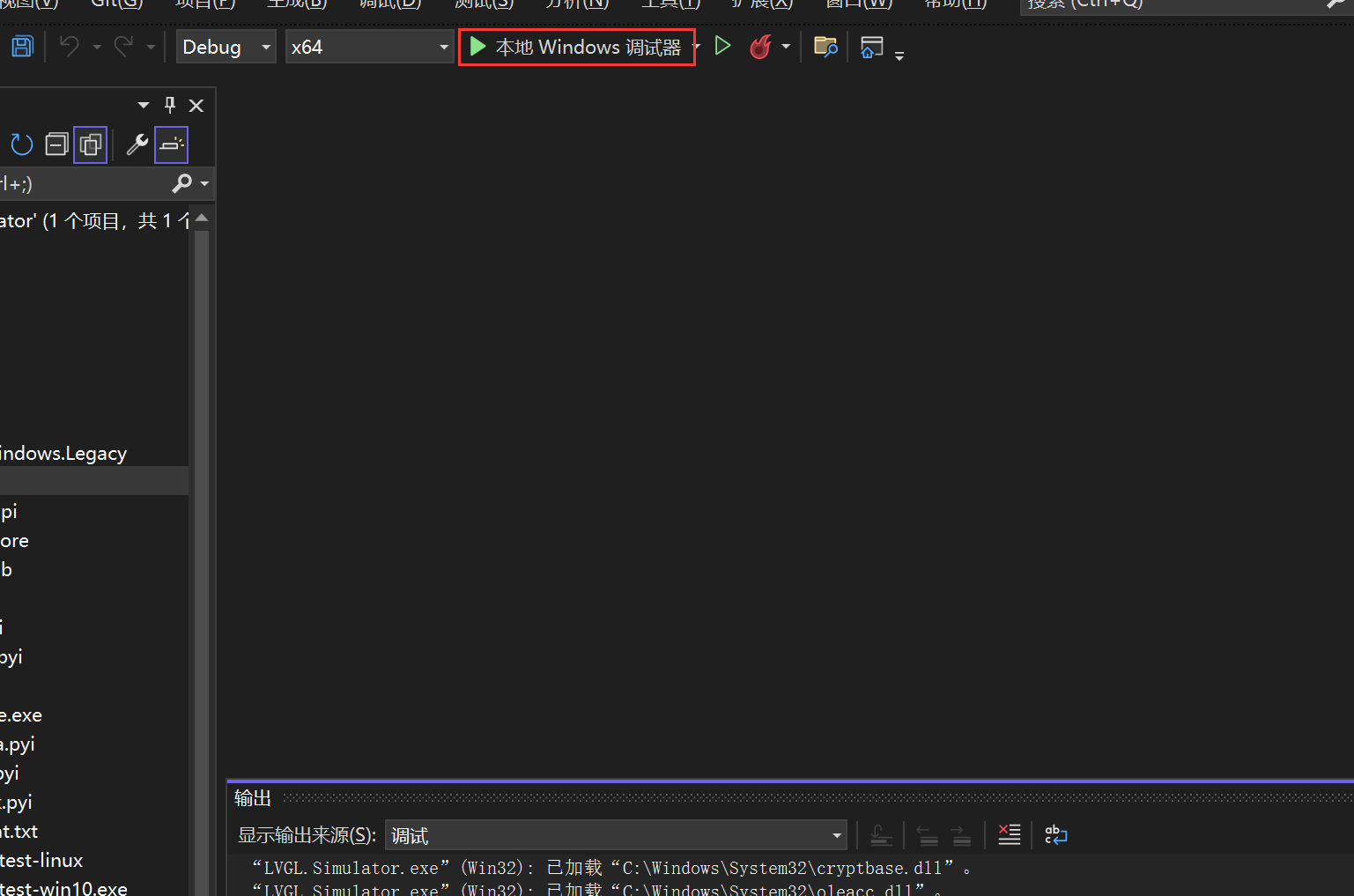In this example you can click the button and then view the output.